# Demo 8 - Using Air Pressure Sensor to Measure Height on VisionFive 2

According to StarFive, developers have successfully used Air Pressure Sensor on VisionFive 2, and the specific instructions are as follows:

1. Preparation

• Development board: VisionFive/VisionFive 2
• Air pressure sensor: BMP180
• DuPont Line: Many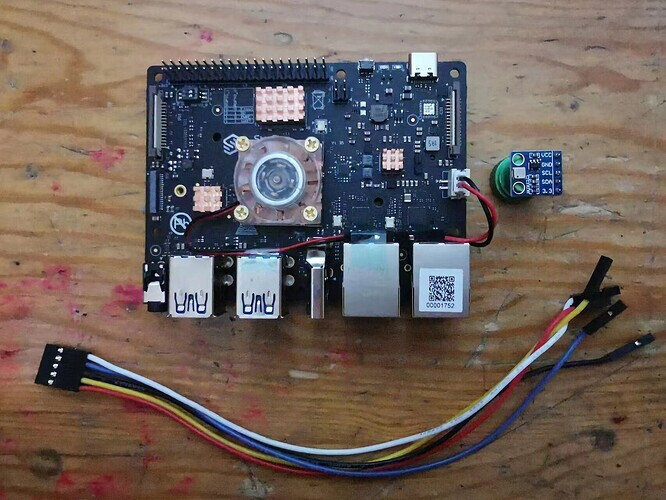2. Principle of air pressure sensor
The specific air pressure sensor modules used in this demo are as follows: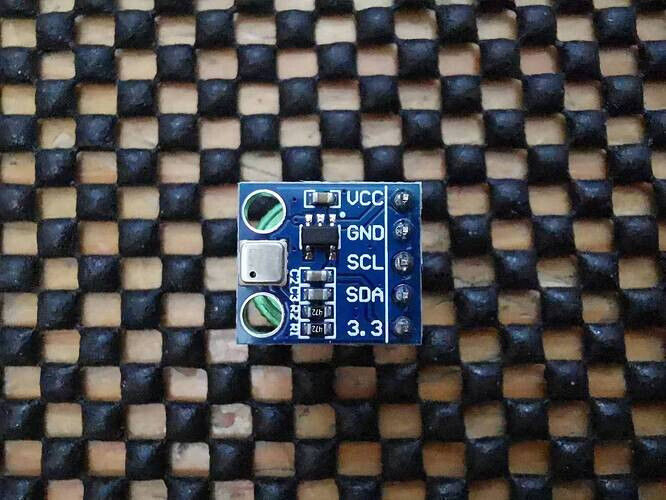The pressure sensor model is BMP180, which is used to measure the absolute pressure of the surrounding air (atmospheric pressure). Its measurement range is from 300hPa - 1100hPa with an accuracy as low as 0.02hPa. It can also measure temperature and obtain height values through conversion formulas.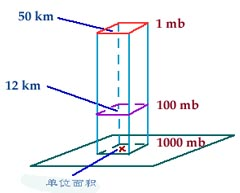The BMP180 air pressure sensor communicates through the I2C interface and can be easily connected to VisionFive. The modules used in this demo can be powered by 5V or 3.3V, but cannot be powered simultaneously.

In practical, influenced by the actual measurement environment, the actual pressure and temperature values measured by BMP180 are relatively accurate, but the calculated altitude is not entirely accurate because air flow, sunlight, and other factors may cause changes in atmospheric pressure and temperature, so the calculated altitude may differ by 0-100 meters. But in a relatively stable environment, reliable data can be obtained by measuring the height difference. For example, if a pressure value is measured on the ground, which is recorded as the basic pressure value, and then measure on different floors based on this basic value, the height value obtained is relatively accurate. For future delays, this method will be used to test the height of the floors.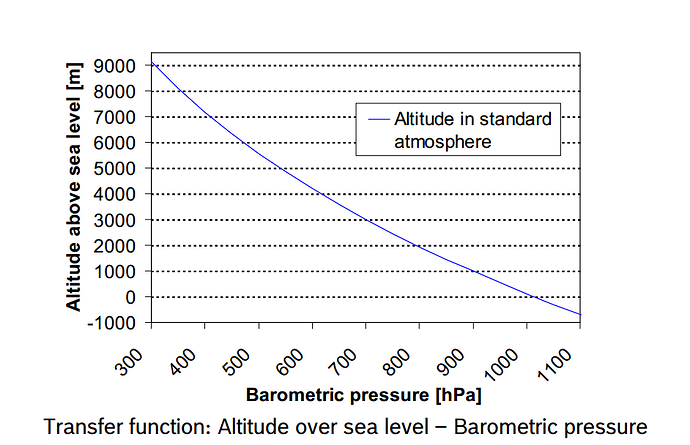3. The use of air pressure sensor
Firstly, refer to the following figure and connect the pressure sensor module to the VisionFive: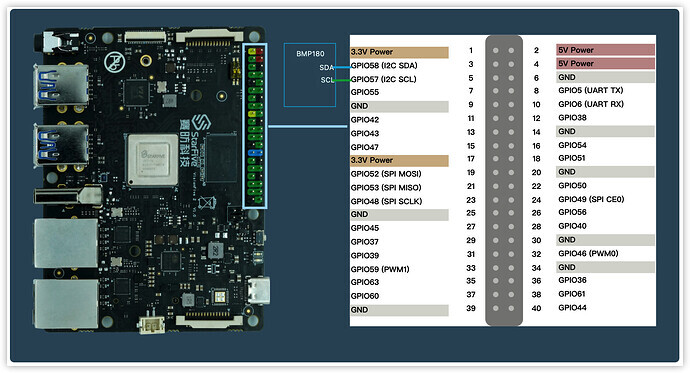Connection: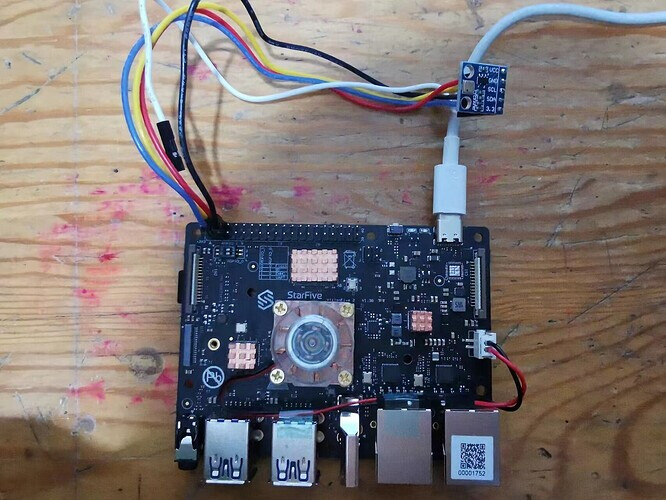Note:
The power supply voltage should be determined based on the actual sensor used. The pressure sensor module used in this demo uses a 5V power supply voltage.

After connecting the BMP180 sensor module to the board, you can use the is2tools to check whether the connection is normal.
Execute following to view the connection status of I2C devices:
`i2cdetect -y -r 0`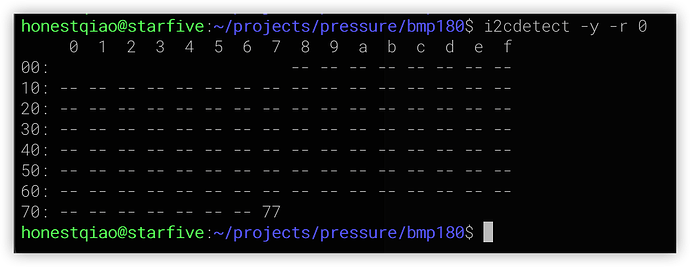From the above figure, it can be seen that the BMP180 sensor module has been successfully connected, and its IIC communication address is 0x77.
To obtain information about BMP180, the board needs to use the IIC interface for communication, which can be assisted by a third-party module bmp180.

``````git clone https://github.com/m-rtijn/bmp180.git
pip install smbus
``````

After downloading the third-party library bmp180, some modifications need to be made to the `bmp180.py` file in order to be used on the board, as follows:

``````"""
This program handles the communication over I2C between a Raspberry Pi and a
BMP180 Temperature/Pressure sensor.
"""

import smbus
import math
from time import sleep

class bmp180:
# Global variables
bus = smbus.SMBus(0) # 星光派开发板40Pin上的I2C使用i2c-0
mode = 1 # TODO: Add a way to change the mode

# BMP180 registers
CONTROL_REG = 0xF4
DATA_REG = 0xF6

# Calibration data registers
CAL_AC1_REG = 0xAA
CAL_AC2_REG = 0xAC
CAL_AC3_REG = 0xAE
CAL_AC4_REG = 0xB0
CAL_AC5_REG = 0xB2
CAL_AC6_REG = 0xB4
CAL_B1_REG = 0xB6
CAL_B2_REG = 0xB8
CAL_MB_REG = 0xBA
CAL_MC_REG = 0xBC
CAL_MD_REG = 0xBE

# Calibration data variables
calAC1 = 0
calAC2 = 0
calAC3 = 0
calAC4 = 0
calAC5 = 0
calAC6 = 0
calB1 = 0
calB2 = 0
calMB = 0
calMC = 0
calMD = 0

# Get the calibration data from the BMP180

# I2C methods

register -- the register to read from.
"""

if msb > 127:
msb -= 256

return (msb << 8) + lsb

Reads the given register and the following, and combines them as an
unsigned 16-bit value.
register -- the register to read from.
"""

return (msb << 8) + lsb

# BMP180 interaction methods

"""Reads and stores the raw calibration data."""

def get_raw_temp(self):
"""Reads and returns the raw temperature data."""
# Write 0x2E to CONTROL_REG to start the measurement

# Wait 4,5 ms
sleep(0.0045)

# Read the raw data from the DATA_REG, 0xF6

# Return the raw data
return raw_data

def get_raw_pressure(self):
"""Reads and returns the raw pressure data."""
# Write appropriate data to sensor to start the measurement
self.bus.write_byte_data(self.address, self.CONTROL_REG, 0x34 + (self.mode << 6))

# Sleep for 8 ms.
# TODO: Way to use the correct wait time for the current mode
sleep(0.008)

raw_data = ((MSB << 16) + (LSB << 8) + XLSB) >> (8 - self.mode)

return raw_data

def get_temp(self):
"""Reads the raw temperature and calculates the actual temperature.

The calculations used to get the actual temperature are from the BMP-180
datasheet.
Returns the actual temperature in degrees Celcius.
"""
UT = self.get_raw_temp()

X1 = 0
X2 = 0
B5 = 0
actual_temp = 0.0

X1 = ((UT - self.calAC6) * self.calAC5) / math.pow(2, 15)
X2 = (self.calMC * math.pow(2, 11)) / (X1 + self.calMD)
B5 = X1 + X2
actual_temp = ((B5 + 8) / math.pow(2, 4)) / 10

return actual_temp

def get_pressure(self):
"""Reads and calculates the actual pressure.

Returns the actual pressure in Pascal.
"""
UP = self.get_raw_pressure()
UT = self.get_raw_temp()
B3 = 0
B4 = 0
B5 = 0
B6 = 0
B7 = 0
X1 = 0
X2 = 0
X3 = 0
pressure = 0

# These calculations are from the BMP180 datasheet, page 15

# Not sure if these calculations should be here, maybe they could be
# removed?
X1 = ((UT - self.calAC6) * self.calAC5) / math.pow(2, 15)
X2 = (self.calMC * math.pow(2, 11)) / (X1 + self.calMD)
B5 = X1 + X2

# Todo: change math.pow cals to constants
B6 = B5 - 4000
X1 = (self.calB2 * (B6 * B6 / math.pow(2, 12))) / math.pow(2, 11)
X2 = self.calAC2 * B6 / math.pow(2, 11)
X3 = X1 + X2
B3 = (((self.calAC1 * 4 + int(X3)) << self.mode) + 2) / 4
X1 = self.calAC3 * B6 / math.pow(2, 13)
X2 = (self.calB1 * (B6 * B6 / math.pow(2, 12))) / math.pow(2, 16)
X3 = ((X1 + X2) + 2) / math.pow(2, 2)
B4 = self.calAC4 * (X3 + 32768) / math.pow(2,15)
B7 = (UP - B3) * (50000 >> self.mode)

if B7 < 0x80000000:
pressure = (B7 * 2) / B4
else:
pressure = (B7 / B4) * 2

X1 = (pressure / math.pow(2, 8)) * (pressure / math.pow(2, 8))
X1 = (X1 * 3038) / math.pow(2, 16)
X2 = (-7357 * pressure) / math.pow(2, 16)
pressure = pressure + (X1 + X2 + 3791) / math.pow(2, 4)

return pressure

def get_altitude(self, sea_level_pressure = 101325):
"""Calulates the altitude.

This method calculates the altitude using the pressure.
This method is not reliable when the sensor is inside.
sea_level_pressure -- the pressure at the sea level closest to you in
Pascal.
Returns the altitude in meters.

!!! This method probably does not work correctly. I've tried to test
it but at the moment I have no way of verifying the data. !!!
"""
altitude = 0.0
pressure = float(self.get_pressure())

# altitude = 44330.0 * (1.0 - math.pow(pressure / sea_level_pressure, 0.00019029495))
altitude = 44330.0 * (1.0 - pow(pressure / sea_level_pressure, (1.0/5.255))) # 矫正计算结果
altitude = round(altitude, 2)

return altitude

if __name__ == "__main__":
bmp = bmp180(0x77)
print(bmp.get_temp())
print(bmp.get_pressure())
print(bmp.get_altitude())
``````

Then, refer to the example and write the following program to read the required data:

``````# -*- coding: utf-8 -*-
from bmp180 import bmp180
import time

bmp = bmp180(0x77)

while True:
temp = bmp.get_temp()
pressure = bmp.get_pressure() / 1000
# altitude = bmp.get_altitude() # 当参数为空时，用于测量基准值
altitude = bmp.get_altitude(102225.9) # 以基准值为基础，测量高度值
print("当前温度: %0.1f ℃" % temp)
print("当前气压: %0.4f kPa" % pressure)
print("可能高度: %0.2f 米\n" % altitude)
time.sleep(2)
``````

Then run `read_data_from_bm180.py` can output the actual measured data.

``````pyhon3 read_data_from_bm180.py
``````

The actual results are as follows: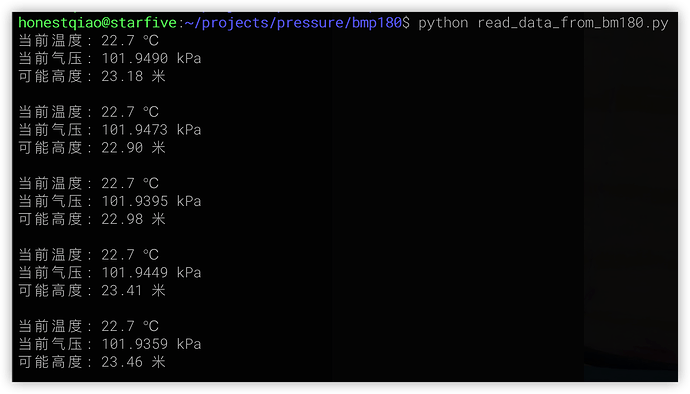In actual use, it is necessary to use `bmp.get_altitude()` to measure the reference value, and then use `bmp.get_altitude(baseline value)` to measure the Elevation value based on this reference value.
In actual measurements, due to environmental influences, the result values may drift to a certain extent. It is possible to consider continuously reading multiple pieces of data and then using certain algorithms to obtain the final reasonable test results.

4. Summary
In this demo, we learned the basic use of air pressure sensors.
In fact, pressure sensors are widely used in indoor and outdoor navigation, weather forecasting, indoor environment monitoring, fan power control and many other occasions.

2 Likes